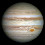## Sunday, 5 July 2015

### Two Dimensional (2D) Image Convolution in CUDA by Shared & Constant Memory: An Optimized way

After learning the concept of two dimension (2D) Convolution and its implementation in C language; the next step is to learn to optimize it. As Convolution is one of the most Compute Intensive task in Image Processing, it is always better to save time required for it. So, today I am going to share a technique to optimize the Convolution process by using CUDA. Here we will use Shared Memory and Constant Memory resources available in CUDA to get fastest implementation of Convolution.

Here is the strategy,
• We will store convolution mask in Constant Memory as all threads will need the same Mask. Constant Memory is the off-chip memory present on GPU card which is read-only memory and faster than Global memory. The Mask is passed to kernel with the keyword "__restrict__". Check for it in the kernel definition.
• Instead of accessing complete image and performing the convolution operation on it; we will fix TILE size. The image pixels are copied to this TILE first and then convolution is performed accordingly. This TILE will be stored in the Shared Memory of GPU card which is on-chip memory and faster than the Global Memory.
Following is the sample code of the implementation. You can also get it from my GitHub repository.

``````
/*
* Title: 2D Image Convolution on GPU by using Shared Memory and Constant Memory.
*
* Image Size: 2048 X 2048
* Mask Size: 64 X 64
* TILE_WIDTH 32
*
*
* */
#include<stdio.h>
#include<cuda_runtime_api.h>
#include<cuda.h>
#include<stdlib.h>
#include<assert.h>

#define wbCheck(stmt)  do {                                                    \
cudaError_t err = stmt;                                               \
if (err != cudaSuccess) {                                             \
printf( "Failed to run stmt %d ", __LINE__);                       \
printf( "Got CUDA error ...  %s ", cudaGetErrorString(err));    \
return -1;                                                        \
}                                                                     \
} while(0)

#define TILE_WIDTH 32  //16 X 16 TILE
#define w_x (TILE_WIDTH + Mask_width - 1) //Shared Memory Elements needed to load as per Mask Size
#define w_y (TILE_WIDTH + Mask_height - 1)
#define clamp(x) (min(max((x), 0.0), 1.0))

//@@ INSERT CODE HERE
__global__ void convolution(float *I, const float* __restrict__ M, float *P,int channels, int width, int height)
{
__shared__ float N_ds[w_y][w_x];
int k;
for (k = 0; k < channels; k++)
{
//1.dest: RMO ID 2. destY & destX: Row and Column of Shared Memory
//3. srcY & srcX: Indexes to fetch data from input Image
//4. src: RMO index of Input Image

destY = dest / w_x, destX = dest % w_x,
src = (srcY * width + srcX) * channels + k;
if (srcY >= 0 && srcY < height && srcX >= 0 && srcX < width)
N_ds[destY][destX] = I[src];
else
N_ds[destY][destX] = 0.0;

for (int iter=1; iter <= (w_x * w_y) / (TILE_WIDTH*TILE_WIDTH); iter++)
{
destY = dest / w_x, destX = dest % w_x;
src = (srcY * width + srcX) * channels + k;
if (destY < w_y && destX < w_x)
{
if (srcY >= 0 && srcY < height && srcX >= 0 && srcX < width)
N_ds[destY][destX] = I[src];
else
N_ds[destY][destX] = 0.0;
}
}

float accum = 0;
int y, x;
for (y = 0; y < Mask_width; y++)
for (x = 0; x < Mask_width; x++)
y = blockIdx.y * TILE_WIDTH + threadIdx.y;
x = blockIdx.x * TILE_WIDTH + threadIdx.x;
if (y < height && x < width)
//P[(y * width + x) * channels + k] = clamp(accum);
P[(y * width + x) * channels + k] = accum;
}
}

float convolution_2D_OnHost(float * N,float * M,int width, int height,int i,int j);

int main() {

int maskRows=Mask_height; // Set it as per requirement of 64 X 32

int imageChannels=1;
int imageWidth=2048;
int imageHeight=2048;

float * hostInputImageData;
float * hostOutputImageData;
float * deviceInputImageData;
float * deviceOutputImageData;
float * outputImageOnHost;

{
}

//Comment this assert code for 64 X 32
//assert(maskRows == 5); /* mask height is fixed to 5 in this mp */
//assert(maskColumns == 5); /* mask width is fixed to 5 in this mp */

//To store Memory
hostInputImageData = (float *) malloc(sizeof(float)*imageWidth*imageHeight);
hostOutputImageData = (float *) malloc(sizeof(float)*imageWidth*imageHeight);
outputImageOnHost = (float *) malloc(sizeof(float)*imageWidth*imageHeight);
for(int i=0;i<imageWidth*imageHeight;i++)//To set Image data as 1.0
{
hostInputImageData[i]=1.0;
}

wbCheck(cudaMalloc((void **) &deviceInputImageData, imageWidth * imageHeight * imageChannels * sizeof(float)));
wbCheck(cudaMalloc((void **) &deviceOutputImageData, imageWidth * imageHeight * imageChannels * sizeof(float)));

wbCheck(cudaMemcpy(deviceInputImageData,
hostInputImageData,
imageWidth * imageHeight * imageChannels * sizeof(float),
cudaMemcpyHostToDevice));
cudaMemcpyHostToDevice));

dim3 dimGrid(((imageWidth-1)/TILE_WIDTH)+1, ((imageHeight-1)/TILE_WIDTH)+1,1);
dim3 dimBlock(TILE_WIDTH, TILE_WIDTH, 1);
imageChannels, imageWidth, imageHeight);

cudaError_t err1 = cudaPeekAtLastError();
printf( "Got CUDA error ... %s \n", cudaGetErrorString(err1));

cudaMemcpy(hostOutputImageData,
deviceOutputImageData,
imageWidth * imageHeight * imageChannels * sizeof(float),
cudaMemcpyDeviceToHost);

//printf("\n Output from Device:\n");
#if 0  //comment this to run the portion of code
for(int i=0;i<imageWidth*imageHeight;i++)
{
if(i>0 && (i%imageWidth==0))
printf("\n");
printf("%0.2f \t",*(hostOutputImageData+i));

}
#endif

//Convolution on Host
for(int i=0;i<imageWidth;i++)
{
for(int j=0;j<imageHeight;j++)
{
}
}

//printf("\n Output from Host:\n");
#if 0  //comment this to run the portion of code
for(int i=0;i<imageWidth*imageHeight;i++)
{
if(i>0 && (i%imageWidth==0))
printf("\n");
printf("%0.2f \t",*(outputImageOnHost+i));

}
#endif

for(int i=0;i<imageWidth*imageHeight;i++)
{
if(outputImageOnHost[i]!=hostOutputImageData[i])
{
printf("\nMismatch at (Row,Col) = [%d][%d], hostComputed[]: %0.0f And device[]: %0.0f", i / imageWidth, i % imageHeight, outputImageOnHost[i], hostOutputImageData[i]);
}
}

cudaFree(deviceInputImageData);
cudaFree(deviceOutputImageData);

free(hostInputImageData);
free(hostOutputImageData);

printf("\n Number of Threads Per Block created in code: %d",TILE_WIDTH*TILE_WIDTH);
printf("\n Number of Blocks Created :%d",(((imageWidth-1)/TILE_WIDTH)+1)*(((imageWidth-1)/TILE_WIDTH)+1));
printf("No Error");
return 0;
}

float convolution_2D_OnHost(float * N,float * M,int width, int height,int i,int j)
{
float Pvalue=0.0;
int N_start_point_i = i - (Mask_width/2);
int N_start_point_j = j - (Mask_height/2);

{
{
if(((N_start_point_i+k)>=0) && ((N_start_point_i+k)<width)&&((N_start_point_j+l)>=0)&&((N_start_point_j+l)<height))
{
}
}
}

// return(clamp(Pvalue));
return((Pvalue));
}

```
```

==>Posted By Yogesh B. Desai

Previous Post: Two Dimensional Image Convolution: A Basic Approach

1.2.